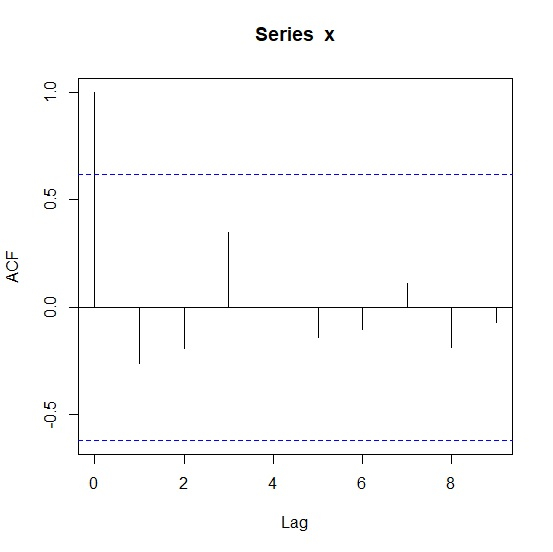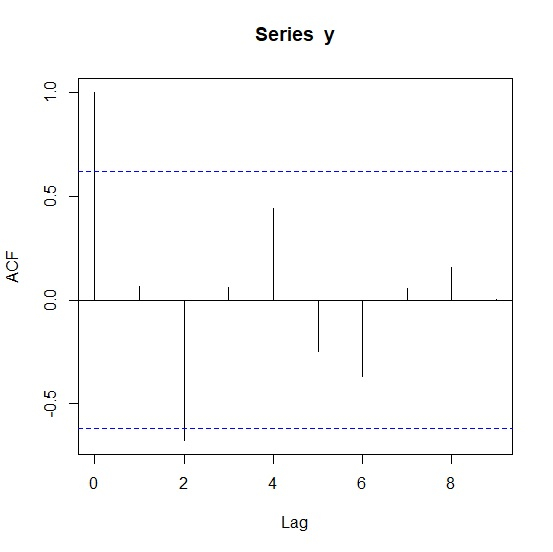# How to create ACF plot in R?

The autocorrelation plot or ACF plot is a display of serial correlation in data that changes over time. The ACF plot can be easily created by using acf function.

For example, if we have a vector called V then we can create its autocorrelation plot by using the command given below −

acf(V)

Check out the below examples to understand how it can be done.

## Example 1

To create ACF plot in R, use the code given below −

x<-sample(1:9,10,replace=TRUE)
x

If you execute the above given code, it generates the following output −

 2 5 6 7 1 7 9 3 9 7


To create ACF plot in R, add the following code to the above snippet −

x<-sample(1:9,10,replace=TRUE)
acf(x)

## Output

If you execute all the above given codes as a single program, it generates the following output −## Example 2

To create ACF plot in R, use the code given below −

y<-rpois(10,5)
y

If you execute the above given code, it generates the following output −

 7 5 2 4 7 3 2 6 8 5


To create ACF plot in R, add the following code to the above snippet −

y<-rpois(10,5)
acf(y)

## Output

If you execute all the above given codes as a single program, it generates the following output −